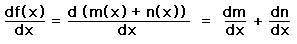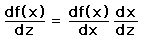# Properties of the Derivative

The derivative has certain special properties when applied to combinations of functions.

## Derivative of the product of two functions

If the function f(x) is a product of two functions m(x) and n(x), then the derivative of this product is## Derivative of the sum of two functions

If the function f(x) is a sum of two functions m(x) and n(x), then the derivative of this sum is simply the sum of the derivatives:## Chain rule for differentiation

If the function y = f(x) and x=g(z), then the derivative of y with respect to z can be written as a product of derivatives:Index

Derivative concepts

 HyperPhysics*****HyperMath*****Calculus R Nave
Go Back